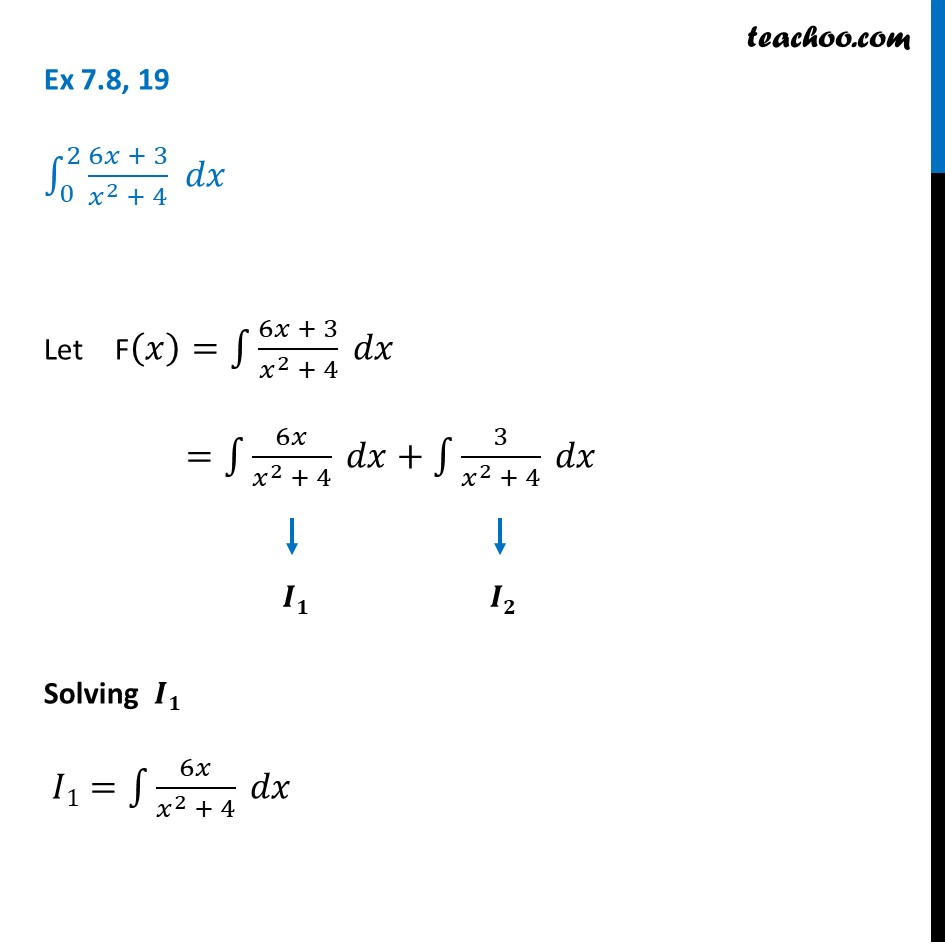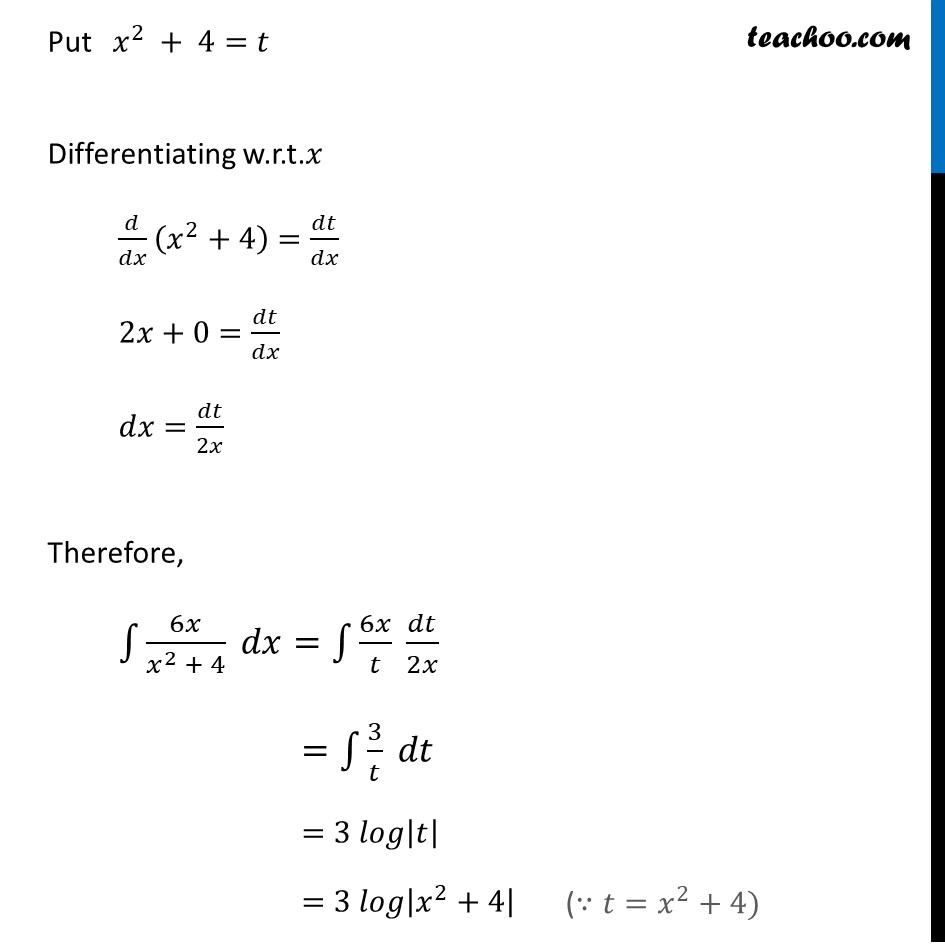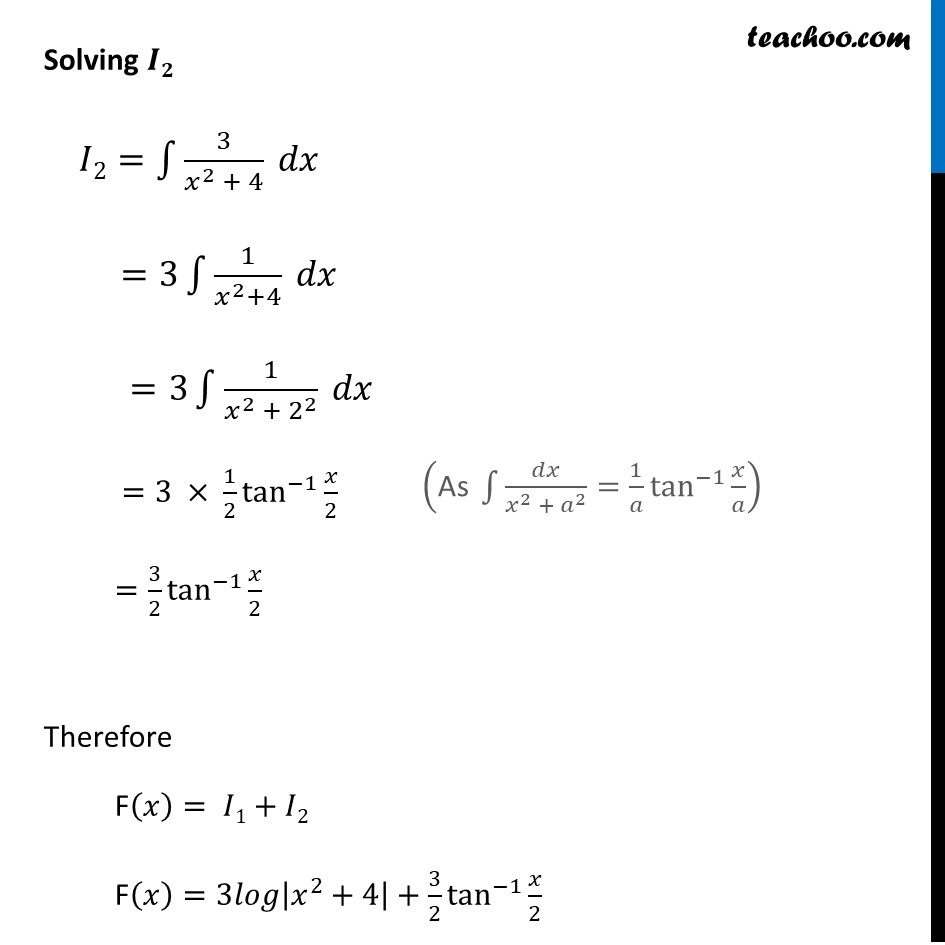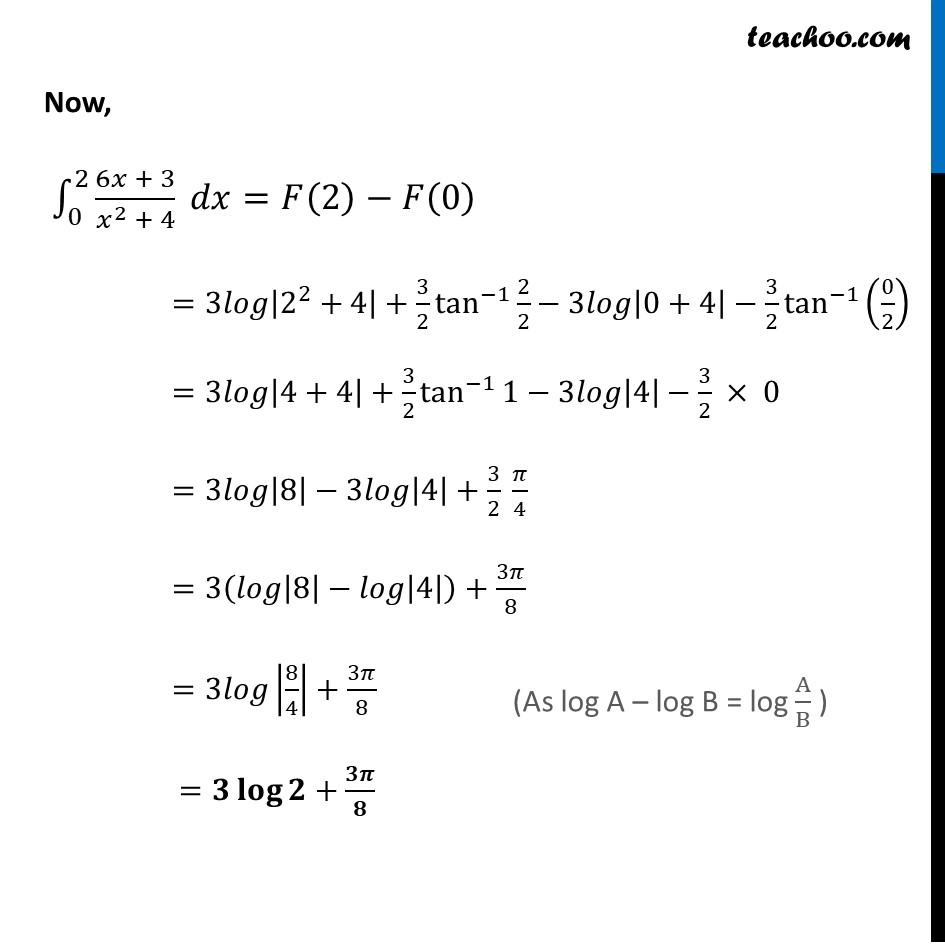Ex 7.8

Chapter 7 Class 12 Integrals
Serial order wiseLearn in your speed, with individual attention - Teachoo Maths 1-on-1 Class

### Transcript

Ex 7.8, 19 ∫_0^2▒(6𝑥 + 3)/(𝑥^2 + 4) 𝑑𝑥 Let F(𝑥)=∫1▒〖(6𝑥 + 3)/(𝑥^2 + 4) 𝑑𝑥〗 =∫1▒〖6𝑥/(𝑥^2 + 4) 𝑑𝑥+∫1▒〖3/(𝑥^2 + 4) 𝑑𝑥 〗〗 Solving 𝑰𝟏 𝐼1=∫1▒〖6𝑥/(𝑥^2 + 4) 𝑑𝑥〗 Put 𝑥^2 + 4=𝑡 Differentiating w.r.t.𝑥 𝑑/𝑑𝑥 (𝑥^2+4)=𝑑𝑡/𝑑𝑥 2𝑥+0=𝑑𝑡/𝑑𝑥 𝑑𝑥=𝑑𝑡/2𝑥 Therefore, ∫1▒〖6𝑥/(𝑥^2 + 4) 𝑑𝑥=∫1▒〖6𝑥/𝑡 𝑑𝑡/2𝑥〗〗 =∫1▒〖3/𝑡 𝑑𝑡〗 =3 𝑙𝑜𝑔|𝑡| =3 𝑙𝑜𝑔|𝑥^2+4| Solving 𝑰𝟐 𝐼2=∫1▒〖3/(𝑥^2 + 4) 𝑑𝑥〗 =3∫1▒1/(𝑥^2+4) 𝑑𝑥 =3∫1▒1/(𝑥^2 + 2^2 ) 𝑑𝑥 =3 × 1/2 tan^(−1)⁡〖𝑥/2〗 =3/2 tan^(−1)⁡〖𝑥/2〗 Therefore F(𝑥)= 𝐼1+𝐼2 F(𝑥)=3𝑙𝑜𝑔|𝑥^2+4|+3/2 tan^(−1)⁡〖𝑥/2〗 Now, ∫_0^2▒〖(6𝑥 + 3)/(𝑥^2 + 4) 𝑑𝑥=𝐹(2)−𝐹(0) 〗 =3𝑙𝑜𝑔|2^2+4|+3/2 tan^(−1)⁡〖2/2−3𝑙𝑜𝑔|0+4|−3/2 tan^(−1)⁡(0/2) 〗 =3𝑙𝑜𝑔|4+4|+3/2 tan^(−1)⁡〖1−3𝑙𝑜𝑔|4|−3/2 × 0〗 =3𝑙𝑜𝑔|8|−3𝑙𝑜𝑔|4|+3/2 𝜋/4 =3(𝑙𝑜𝑔|8|−𝑙𝑜𝑔|4|)+3𝜋/8 =3𝑙𝑜𝑔|8/4|+3𝜋/8 =𝟑 𝐥𝐨𝐠⁡〖𝟐+𝟑𝝅/𝟖〗